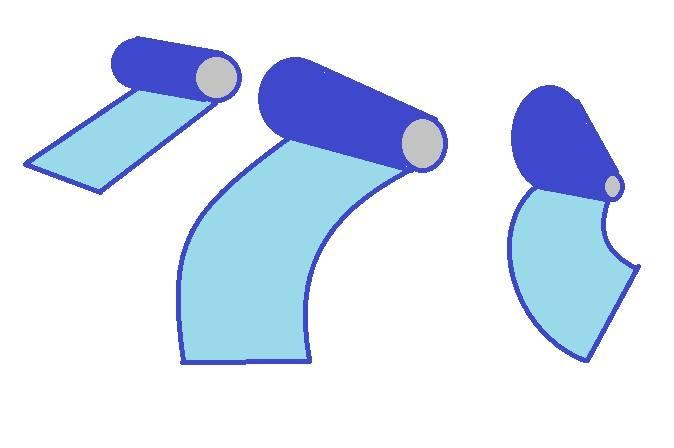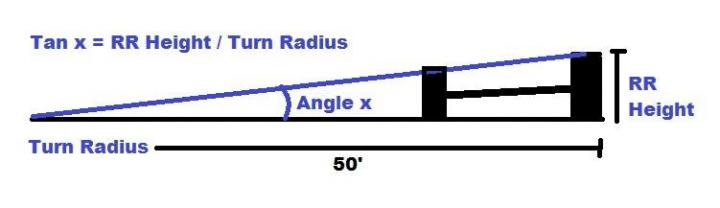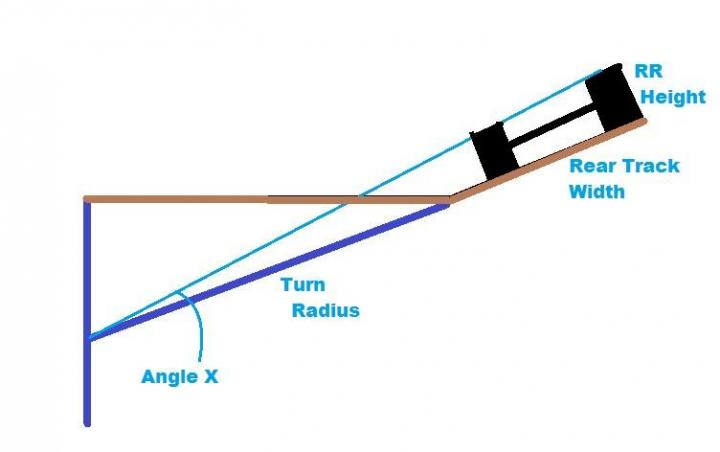Articles Home » Technical Articles » Understanding and Calculating Stagger

## Understanding and Calculating Stagger

### Article

Most of us who have raced oval tracks, and karting in particular, have discussed and tried various setups to go faster.  One of the most basic tuning tools we have is the concept of "Stagger".  Simply put, stagger is the difference in diameter between the outside tire and it's corresponding inside tire.  (For the Americas, with typical Left Turn Only (LTO) karts, the right tire is "outside", left side tire is "inside")

The principle reason that we use stagger instead of equal diameter tires is that we are seeking to assist the kart in naturally turning left.  Karts, having a solid axle, are naturally inclined to "scrub" either the inside tire or the outside tire when the driver attempts to initiate a turn by rotating the front wheels.  If there is no stagger, the maximum scrub condition exists -- the front tires must fight the grip in the scrubbing rear tire in order to rotate the kart.  This scrubbing results in wasted friction in the form of heat, and also resists the forward motion of the kart.  Obviously this is a bad thing when trying to maximize our speed.  To retain our speed while cornering, then, we introduce varying amounts of stagger to induce a turn with little or no driver effort.We can see from the diagram that the cylindrical column (equal to a kart with zero stagger) will tend to go straight, while a kart with stagger (cone) will tend to turn naturally.  The steeper the angle created by stagger, the tighter the radius of the turn will be.

So how do we find optimum stagger?

If karts raced in a perfect circle, we could use geometry to calculate the "ideal" stagger.  First we'd need to know the radius of the turn.  For easy calculation, let's assume the radius is 50 feet to our ideal line.  Secondly, we need the diameter of the outside tire.  Since most kart tires roll out around 34", we know that the diameter = 34 / 3.1415, or approximately 10.82".

Since we set the radius of the turn at 50', we can calculate the angle between the center point of the turn and the top of the outside tire.  From our high school trig, we know that the TANgent of the base angle in a right triangle is equal to it's OPPosite side divided by it's ADJacent side.  In our example, the tangent of this angle is equal to (10.82" / 50').Converting the 50' to inches, we determine that the TANgent of the angle = (10.82/600) or 0.018.  We still need to find the height of the inside tire to get its' diameter and stagger.  Our problem is a bit easier because we can set another right triangle inside the first.  The difference is the width of our rear track.

Since we now know the TANgent of the original angle, we can use geometry back in the other direction to find the height of the inside tire.  Let's assume our rear track width is 43" from the center of the outside tire to the center of the inside tire.  For our new triangle, the radius of the turn becomes 50' - 43" or 600"-43" = 557".  We put this back into the formula above:

TAN 0.000029 = ( x / 557) where x is the diameter of our inside tire.

Rearranging and solving for x, we see that  the diameter is (0.018) * 557"= 10.026".  And now that we know the diameter, we multiply by 3.1415 (pi) to get the circumference of 31.5".

So, in this case, where we have a track with a 50' radius circle,  and a rear track of 43" with a 34" RR rollout, the formula indicates a rollout of 31.5" on the LR and a stagger of 2.5".  Obviously, this is a simplified case, but the same math works for getting a baseline guesstimate for your local track.

We must consider, however, that we're not all racing on circles.  For most tracks, we'll end up driving a line that looks more like an ellipse.  That means that if set the kart up with the "ideal" stagger determined by the turn radius, we'll be wasting some energy trying to keep the kart from turning at every point other than the apex.  Not good.  So, we must compromise.  We can calculate the ratio of time turning to time going straight.  And using this ratio, we can adjust the stagger upward for tracks that are turn-dominated and lessen stagger for paper clip tracks with long straights.

The ultimate stagger you end up running will likely be found by experiment, but this method will give you a starting point for unfamiliar tracks.  One other thing to keep in mind is that this baseline doesn't account for banked, grippy tracks.  Such tracks will tend to provide more relative bite, so less stagger can be utilized, scrubbing less speed off on the straights.  The geometry is a bit more complicated for such cases since the triangle drawn is skewed, but the calculation method is the same.  (Notice in the diagram below that we're extending the centerline of the turn,  Where the banked track would intersect is underground, resulting in a shallower angle, therefore a shallower stagger.)I hope that this has helped clarify where stagger numbers come from and how to guess what your setup should be when no factory rep is available...  Feel free to shoot me any questions or comments to "[email protected]

~Ted Hamilton "The Faster Pastor"